# Fréchet variation

(diff) ← Older revision | Latest revision (diff) | Newer revision → (diff)

One of the numerical characteristics of a function of several variables that can be regarded as a multi-dimensional analogue of the variation of a function of a single variable. Suppose that a real-valued function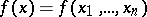is given on the-dimensional parallelopipedon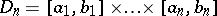and introduce the notation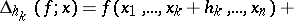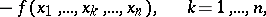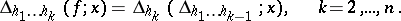Letbe an arbitrary partition of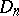by hyperplanes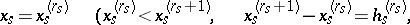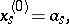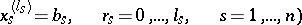into-dimensional parallelopipeda, and let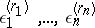take the values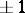in an arbitrary way. The Fréchet variation is defined as follows: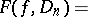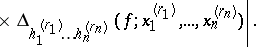If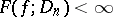, then one says thathas bounded (finite) Fréchet variation on, and the class of all such functions is denoted by. For, this class was introduced by M. Fréchet  in connection with the investigation of the general form of a bilinear continuous functional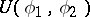on the space of functions of the form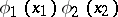that are continuous on the square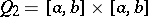. He proved that every such functional can be represented in the form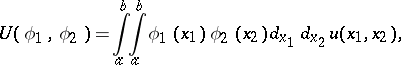where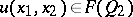,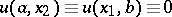.

Analogues of many of the classical criteria for the convergence of Fourier series are valid for-periodic functions in the class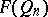(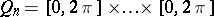, see ). For example, if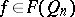,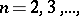then the rectangular partial sums of the Fourier series ofconverge at every point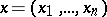to the number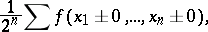where the summation is taken over all thepossible combinations of the signs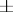. Here, if the function is continuous, the convergence is uniform (an analogue of the Jordan criterion).